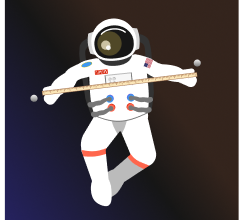# The Strength of Gravity

Newton proposed that gravity is a universal force, acting between any pairs of masses, large or small. This claim must be tested.

If you were tasked with making a measurement of gravity between two non-astronomical objects, you might expect it would be easiest to make your measurement away from the influence of other massive bodies. For this quiz, imagine you are in deep space, far away from all mass except two metal spheres with masses $$m_1$$ and $$m_2$$.By considering the force between the spheres

$F=\frac{Gm_1 m_2}{r^2}$

where $$G=\SI[per-mode=symbol]{6.67e-11}{\meter\cubed\per\kilo\gram\per\second\squared},$$ we can explore how the strength of gravity is encoded in Newton's gravitational law, and how gravity's long-range influence arises.

# The Strength of GravityIf the two spheres are $$\SI{1}{\meter}$$ apart, and both have mass $$\SI{1}{\kilo\gram}$$, you would not want your measurement device to display forces, for example, in giganewtons ($$\SI{1}{\giga\newton}=\SI{10^9}{\newton}$$). This scale would be more appropriate for gravity between planet-sized masses.

What scale (in $$\si{N}$$) do you expect would best describe your measurement of the gravitational force the spheres apply on each other?

# The Strength of Gravity

We introduced Newton's constant $$G$$ in a previous quiz as a parameter to fit in Newton's model of gravity. Now we see that $$G$$ sets the universal scale of gravitational interactions between any pair of objects.

Now that you've calibrated your force meter, you are ready to measure the gravitational force between the $$\SI{1}{\kilo\gram}$$ masses. But there's a problem: your body mass applies a force on the spheres that interferes with your precision measurement of their interaction.

How far away do you have to be from the spheres so that your body mass does not interfere with the experiment?

Details & Assumptions

• You move far enough away that the gravitational force you apply on one of the spheres is less than $$1/10$$ of the force the spheres apply on each other.
• Your mass is $$\SI{50}{\kilo\gram},$$ and assume you can treat yourself approximately as a point mass.

# The Strength of Gravity

Because gravitational forces decrease slowly with distance, large masses that are far away can have significant gravitational influence.

About how far away does the sphere experiment have to be from the Earth so that the Earth's gravitational pull is less than $$1/10$$ of the force the spheres apply on each other?

Details

• The mass of the Earth is $$\SI{5.97\times 10^{24}}{\kilo\gram}$$

# The Strength of Gravity

According to the previous problem, you must perform your experiment at a distance from Earth greater than the distance from the Sun to Neptune. Of course, the Sun and other more massive planets in our solar system exert gravitational forces also.

In fact, the nearest place where the force between the spheres, placed $$\SI{1}{\meter}$$ apart, would be the dominant gravitational influence is outside the solar system, about $$\SI{4.5\times 10^{15}}{\meter}$$ from the Sun. This distance is about $$1/10$$ of the distance to the closest star, Alpha Centauri.

It may seem like an impossible task to design a sensitive experiment to measure the force between two non-astronomical objects, but in the next chapter we will explore the ingenious Cavendish experiment, as well as various other gravitational effects observed on Earth, including

• tides,
• black holes,
• and gravitational redshift.

See you there!

## The Strength of Gravity

### Introduction to Gravity

×

Problem Loading...

Note Loading...

Set Loading...